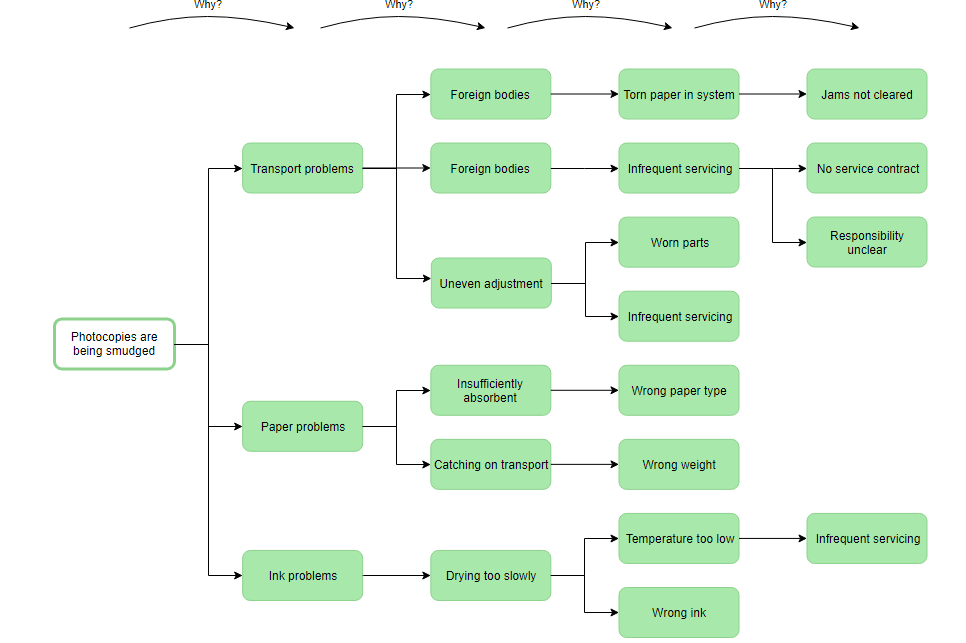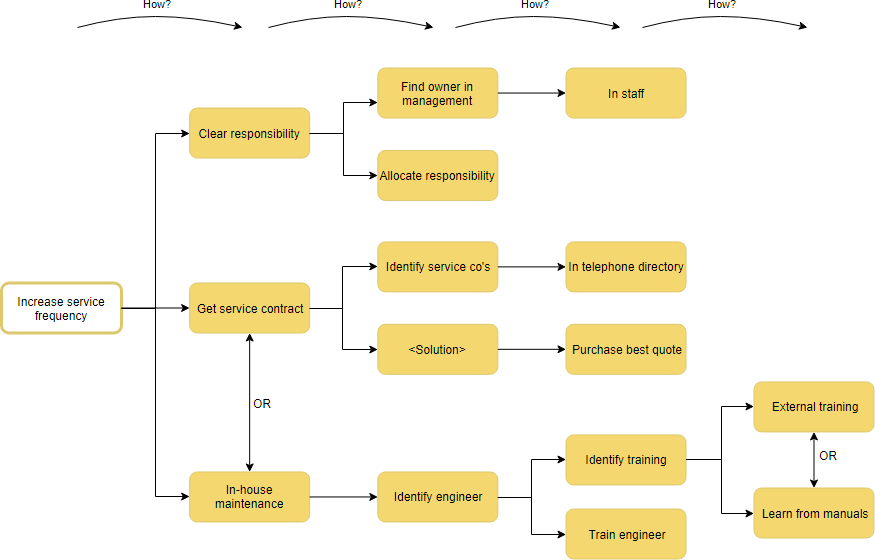Suppose, we have performed the Why-Why analysis to identify the root cause of the problem for the photocopier issue. Once you have discovered why a problem occurs, you then need to find a permanent solution to the problem and this can be easily done by performing the How-How Analysis.Edit this Why-Why Diagram Example

## Solving Why-Why Root Cause with How-How Diagram

Once you have discovered why a problem occurs, the matching question that is asked next is “How?” Given the cause of a problem, the requirement then is to find a solution that will permanently fix the cause. Now, the team attempts to focus on the root causes “Infrequent Servicing” and use the how-how diagram properly fix the problem rather than applying a temporary bandage.

The How-How Diagram works by repeatedly asking the same question of a problem, breaking down the solution into more and more explicit elements. At each stage, there can be multiple answers to the “How” questions, which results in a hierarchical tree-structure. The benefits of How-How Diagram as follows:

1. It allows a group of people to share the mental model of the situation and hence work more harmoniously on it.
2. It allows the re-examination of parts of the analysis, so you can change, remove or add to it at any time. This supports the non-linear way in which we tend to think.
3. It allows you to consciously not to follow some paths, digging only into the most likely areas.

## Four Steps for Creating How-How Diagram

The How-How Diagram is used when seeking a practical solution to a problem. It works by repeatedly asking: ‘How can this do this? ‘. It provides an effective structure for organizing and sequencing possible options as the steps described as following:

1. Write the problem on a Post-it Note and place it to the left of a large work area on the wall. A big sheet of brown paper or several flip-chart pages taped together can help. Note that the starting problem of a How-How session may be the key cause as identified by a preceding Why-Why analysis.
2. Ask is the problem may be solved? Put the name of the root cause as the root of the how-how tree you are trying to solve in the diagram.
3. Repeat this sequence of breaking down the problem once more, this time simply asking “How can I do this?” and keep asking How until you have a root cause or a complete solution.
4. When you have completed the analysis, discuss and identify the key solution to implement.Edit this How-How Diagram Example